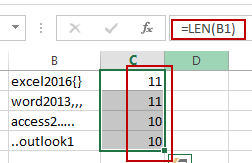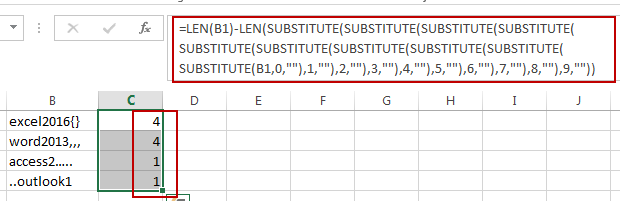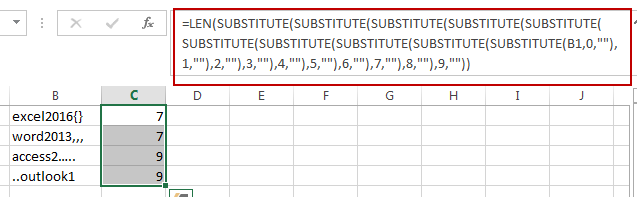# How to Count the Number of Letters or Numbers separately in a Cell in Excel

This post will guide you how to count the number of a given character in a cell in Excel. How do I count only numbers within a single cell excluding all letters and other characters with a formula in Excel. How to count the number of letters excluding all numbers in a given string in Excel.

## Count Total Characters in a Cell

Assuming that you have a list of data in range B1:B4, and you want to count the total number of all characters in one cell, you can use a formula based on the LEN function to get it. Like this:

`=LEN(B1)`

Type this formula in cell C1 and press Enter key on your keyboard, and drag the AutoFill Handle to copy this formula from Cell C1 to range C2:C4.You should notice that the number of all characters in each cell is calculated.

## Count Only Numbers in a Cell

If you want only count the total numbers in cells, excluding letters and other specific characters, you can use a formula based on the LEN function and the SUBSTITUTE function. Like this:

`=LEN(B1)-LEN(SUBSTITUTE(SUBSTITUTE(SUBSTITUTE(SUBSTITUTE(SUBSTITUTE(SUBSTITUTE(SUBSTITUTE(SUBSTITUTE(SUBSTITUTE(SUBSTITUTE(B1,0,""),1,""),2,""),3,""),4,""),5,""),6,""),7,""),8,""),9,""))`

Type this formula into a blank cell and press Enter key. And then drag the AutoFill Handle down to other cells to apply this formula.## Count Only Letters or Other Characters Excluding Numbers

If you want to count only letters and other specific characters in Cells, you can also use another formula based on the LEN function and the SUBSTITUTE function to achieve the result. Like this:

`=LEN(SUBSTITUTE(SUBSTITUTE(SUBSTITUTE(SUBSTITUTE(SUBSTITUTE(SUBSTITUTE(SUBSTITUTE(SUBSTITUTE(SUBSTITUTE(SUBSTITUTE(B1,0,""),1,""),2,""),3,""),4,""),5,""),6,""),7,""),8,""),9,""))`

Type this formula into a blank cell and press Enter key. And then drag the AutoFill Handle down to other cells to apply this formula.### Related Functions

• Excel Substitute function
The Excel SUBSTITUTE function replaces a new text string for an old text string in a text string.The syntax of the SUBSTITUTE function is as below:= SUBSTITUTE  (text, old_text, new_text,[instance_num])….
• Excel LEN function
The Excel LEN function returns the length of a text string (the number of characters in a text string).The syntax of the LEN function is as below:= LEN(text)…

Related Posts

Find and Replace Multiple Values

This post will guide you how to find and replace multiple values at once with VBA macro or using formula  in Excel. How do I make multiple find and replace in Excel. Suppose that you have a few cells containing ...

Extract Last Two Words From Multiple Cells

Just assume that you have a few cells containing values/words and you want to extract the last two words from each cell into another separate cell; then you might think that it's not a big deal; because you would prefer ...

Extract Multiple Lines From A Cell

Suppose that you have listed some text in a single cell which is separated by the line break(you can do it by pressing ALT + ENTER after entering the text), and now you want to extract multiple lines of text ...

How to Remove All Asterisk Characters from Cells in Excel

Sometimes when we opening an excel spreadsheet to check data, we may notice that there are some asterisks exist in texts for security or other reasons. If you feel these special characters are invalid you can remove them from cells. ...

How to Convert Date Format from Dot MM.DD.YYYY to Slash MM/DD/YYYY in Excel

We often enter dates in excel tables, and we can enter current date by quickly press ‘Ctrl+;’ simultaneously. If in some cases we enter a date format that is not what we want, we have to switch the date format ...

How to Remove Quotes for Text or Strings in Excel

When we importing data from other source into Excel we may import quotes or quotations from initial file. And we want to remove these quotes from current file, if we remove them one by one it needs a lot of ...

How to Remove Specific Character from Text Cell in Excel

This post will guide you how to remove a specific character from text cell in Excel. How do I remove certain character from a cell with a formula in Excel 2013/2016. Remove Specific Character Using Find and Replace function Remove ...

How to Count Comma Separated Value in One Cell in Excel

This post will guide you how to count values in a single cell separated by commas with a formula in Excel. How do I count comma separated values in one cell in Excel 2013/2016. Is it possible to have a ...

How to Remove the First/Last Word from Text string in Cell

This post will guide you how to remove the first and the last word from a text string in cells in Excel. How do I use a formula to remove first and last word of a text string in Excel. ...

How to Remove Line Breaks (Carriage Returns) in Excel

This post will explain that how to remove line breaks in your data set in Excel. How do I remove carriage returns or line breaks with a formula in Excel. How to remove line breaks with VBA Macro in Excel. ...

Sidebar